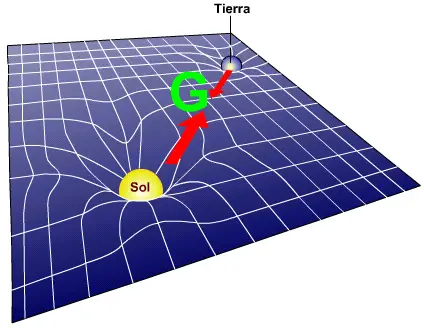# Force of Gravity: Definition, Formula and ExamplesThe force of gravity is a concept that has fascinated humanity for centuries. From an apple falling on Isaac Newton's head to Albert Einstein's formulation of the theory of relativity, gravity has been a fundamental pillar in physics that has shaped our understanding of the universe.

## What Is the Force of Gravity?

The force of gravity is one of the four fundamental forces of nature, along with the electromagnetic force, the strong nuclear force, and the weak nuclear force.

This force is responsible for the mutual attraction between two objects with mass. In simpler terms, it is what makes us fall when we jump and what keeps the Moon in orbit around the Earth.

## Formula: the Law of Universal Gravitation

Gravity is commonly described with Newton's law of universal gravitation, which states that the force of attraction between two objects is directly proportional to the product of their masses and inversely proportional to the square of the distance between them. This law is expressed by the following equation:Where:

• F is the force of gravity between the two objects.
• G is the universal gravitational constant, a fundamental constant in physics.
• m 1 and m 2 are the masses of the two objects.
• r is the distance between the centers of mass of the objects.

This equation shows us that the force of gravity decreases as the distance between objects increases and increases as the mass of the objects increases.

In other words, the heavier an object is and the closer it is to another object, the greater the force of gravity between them.

## The Origin of the Force of Gravity

The fundamental question is: why does the force of gravity exist? The answer is found in Albert Einstein's theory of general relativity within relativistic physics.According to this theory, gravity is due to the curvature of space-time caused by the presence of mass and energy. In other words, massive objects like the Earth curve spacetime around them, and this curvature is what causes other objects to be attracted to them.

Imagine a large object, like Earth, placing a sort of "well" in space-time around it. Smaller objects, such as an apple, will move along the curvature of this well, giving the sensation that they are being pulled towards the Earth.

This geometric description of gravity is a revolutionary way of understanding this force and has been confirmed by numerous experiments and observations.

## Difference Between Weight and Force of Gravity

Weight and the force of gravity are two interconnected concepts but with key differences in physics.

Weight, which in physics is a fundamental concept in the study of dynamics, refers to the gravitational force that acts on an object due to its mass at a specific location, such as the Earth's surface. It is measured in units of force, such as newtons or pound-force, and depends on the mass of the object and the strength of the local gravitational field. On Earth, the acceleration due to gravity is approximately

9.81 m/s^2, so the weight is calculated by multiplying the mass of the object by this acceleration.

On the other hand, the force of gravity is a more general description of the gravitational attraction between two objects with mass, anywhere in the universe. This force is also measured in newtons and is described by Newton's law of universal gravitation, which considers the mass of both objects and the distance that separates them.

## Examples of Gravitational Force in Our Daily LivesThe gravitational force plays a fundamental role in our daily lives, and its influence is evident in a variety of situations.

Here are some examples:

• Falling objects: Gravity is the reason why objects fall when we drop them. From a pen to a hammer, all objects fall due to the Earth's gravitational pull.
• Tides: The gravitational force is also responsible for the tides in the oceans. The Moon exerts a gravitational force on the Earth, which causes the periodic rise and fall of water in the oceans.
• Atmospheric fluidity: Gravity is also responsible for atmospheric pressure, as the mass of the atmosphere is attracted towards the Earth. This pressure is crucial so that the air we breathe does not escape outside the universe and so that it maintains an ideal density to be breathable.

## Gravity in the Cosmos

The influence of gravity is not limited to our planet; It is a dominant force throughout the universe. In astronomy, the gravitational force is crucial to understanding the movement and position of celestial bodies.Here are some examples of its role in the cosmos:

• The solar system: Gravity is fundamental in the formation and stability of the solar system. It keeps the planets in orbit around the Sun and is responsible for the formation of structures such as Saturn's rings.
• Black Holes: Black holes are regions of space with gravity so intense that nothing, not even light, can escape their pull. The formation of black holes is due to the gravitational collapse of massive stars.
• Galaxies: The force of gravity also plays an important role in the formation and evolution of galaxies. The gravitational attraction between stars and dark matter in a galaxy holds the galaxy's structure together.
• Expansion of the universe: Gravity is a key factor in the expansion of the universe.
Author:

Published: October 16, 2023
Last review: October 16, 2023